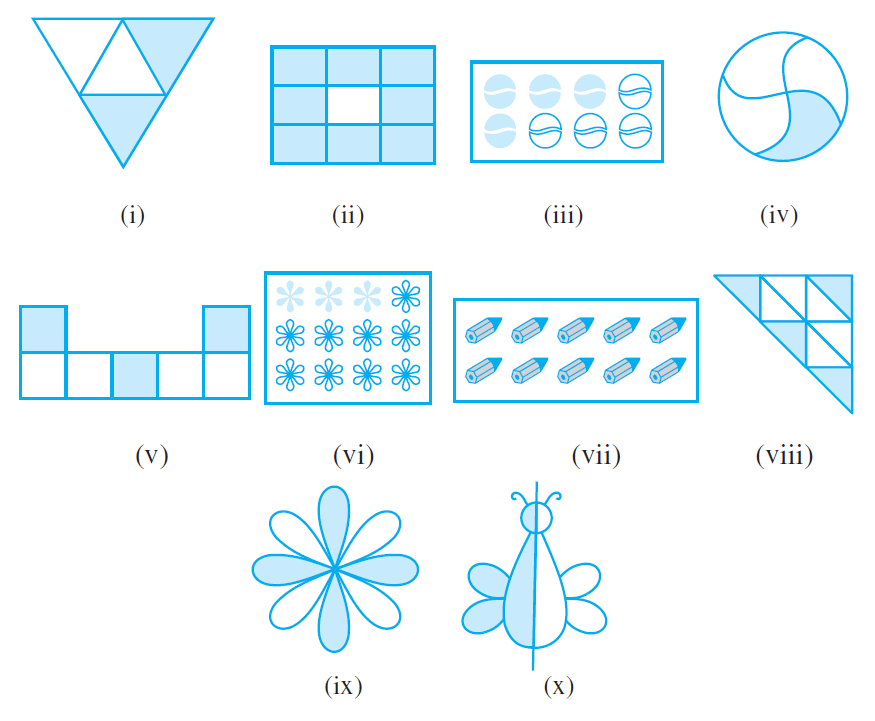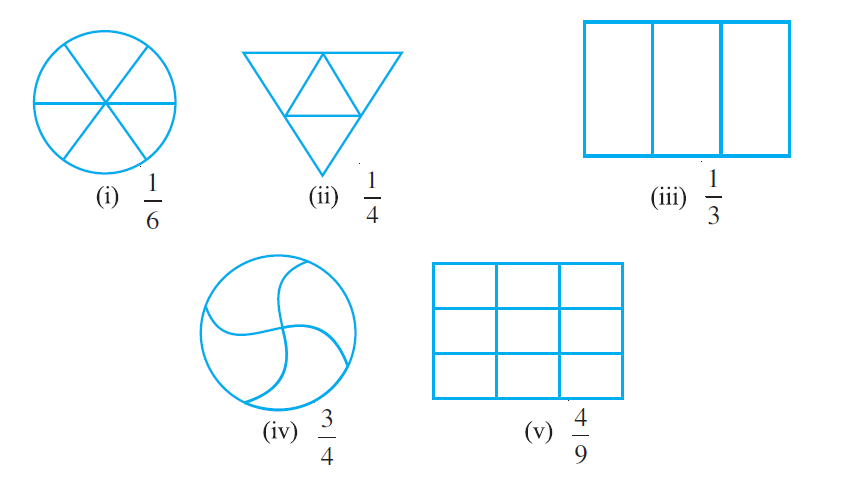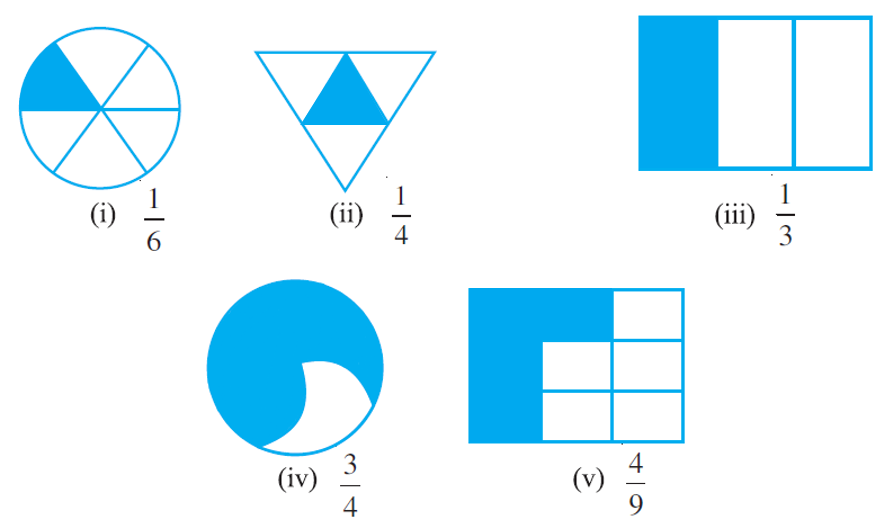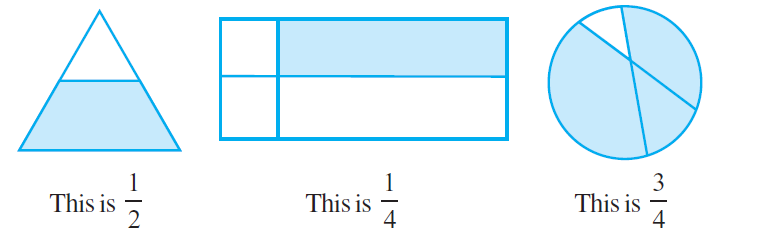# vs.eyeandcontacts.com

## Chapter 7 Fractions Exercise 7.1

Question 1 Write the fraction representing the shaded portion.i)
Total parts = 4
= 2/4 or 1/2

ii)
Total parts = 9
= 8/9

iii)
Total parts = 8
= 4/8 or 1/2

iv)
Total parts = 4
= 1/4

v)
Total parts = 7
= 3/7

vi)
Total parts = 12
= 3/12 or 1/4

vii)
Total parts = 10
= 10/10 or 1

viii)
Total parts = 9
= 4/9

ix)
Total parts = 8
= 4/8 or 1/2

x)
Total parts = 2
= 1/2

Question 2: Colour the part according to the given fraction.Question 3: Identify the error, if any.Question 4: What fraction of a day is 8 hours?

Number of hours in a day = 24 hours
Fraction of a day is 8 hours = ?

= 8/24
= 1/3

Therefore, 1/3 day represents 8 hours.

Question 5: What fraction of an hour is 40 minutes?

Number of minutes in an hour = 60 minutes
Fraction of an hour is 40 minutes = ?

= 40/60
= 1/3

Therefore, 1/3 hour represents 40 minutes.

Question 6: Arya, Abhimanyu, and Vivek shared lunch. Arya has brought two sandwiches, one made of vegetable and one of jam. The other two boys forgot to bring their lunch. Arya agreed to share his sandwiches so that each person will have an equal share of each sandwich.
a) How can Arya divide his sandwiches so that each person has an equal share?
b) What part of a sandwich will each boy receive?

a) Arya will divide each sandwich into three equal parts, and give one part of each sandwich to each one of them.

b) Each boy will receive 1/3 part of a sandwich.

Question 7: Kanchan dyes dresses. She had to dye 30 dresses. She has so far finished 20 dresses. What fraction of dresses has she finished?

Dresses to be dyed by Kanchan = 30
Dresses finished dying by Kanchan = 20
Fraction of dresses she finished = ?

= 20/30
= 2/3

Therefore, she finished 2/3 of dresses.

Question 8: Write the natural numbers from 2 to 12. What fraction of them are prime numbers?

Natural numbers from 2 to 12 = 2, 3, 4, 5, 6, 7, 8, 9, 10, 11, 12 (11)
Prime numbers from 2 to 12 = 2, 3, 5, 7, 11 (5)
Fraction of natural numbers which are prime numbers = ?

= 5/11

Therefore, 5/11 of the natural numbers are prime numbers.

Question 9: Write the natural numbers from 102 to 113. What fraction of them are prime numbers?

Natural number from 102 to 113 = 102, 103, 104, 105, 106, 107, 108, 109, 110, 111, 112, 113 (12)
Prime number from 102 to 113 = 103, 107, 109, 113
Fraction of natural numbers which are prime numbers = ?

= 4/12
= 1/3

Question 10: What fraction of these circles have X’s in them?Total parts = 8
Shaded parts (Circles having X’s in them) = 4
Fraction = Shaded parts (Circles having X’s in them)/Total parts

= 4/8 or 1/2

Therefore, 1/2 or 4/8 of the fraction of the circles have X’s in them.

Question 11: Kristin received a CD player for her birthday. She bought 3 CDs and received 5 others as gifts. What fraction of her total CDs did she buy and what fraction did she receive as gifts?

Number of CDs Kristin brought = 3
Total CDs she have = 5 + 3 = 8

Fraction of CDs she brought = ?
= Number of CDs Kristin brought/Total CDs she have
= 3/8